# Solid State Chemistry Numericals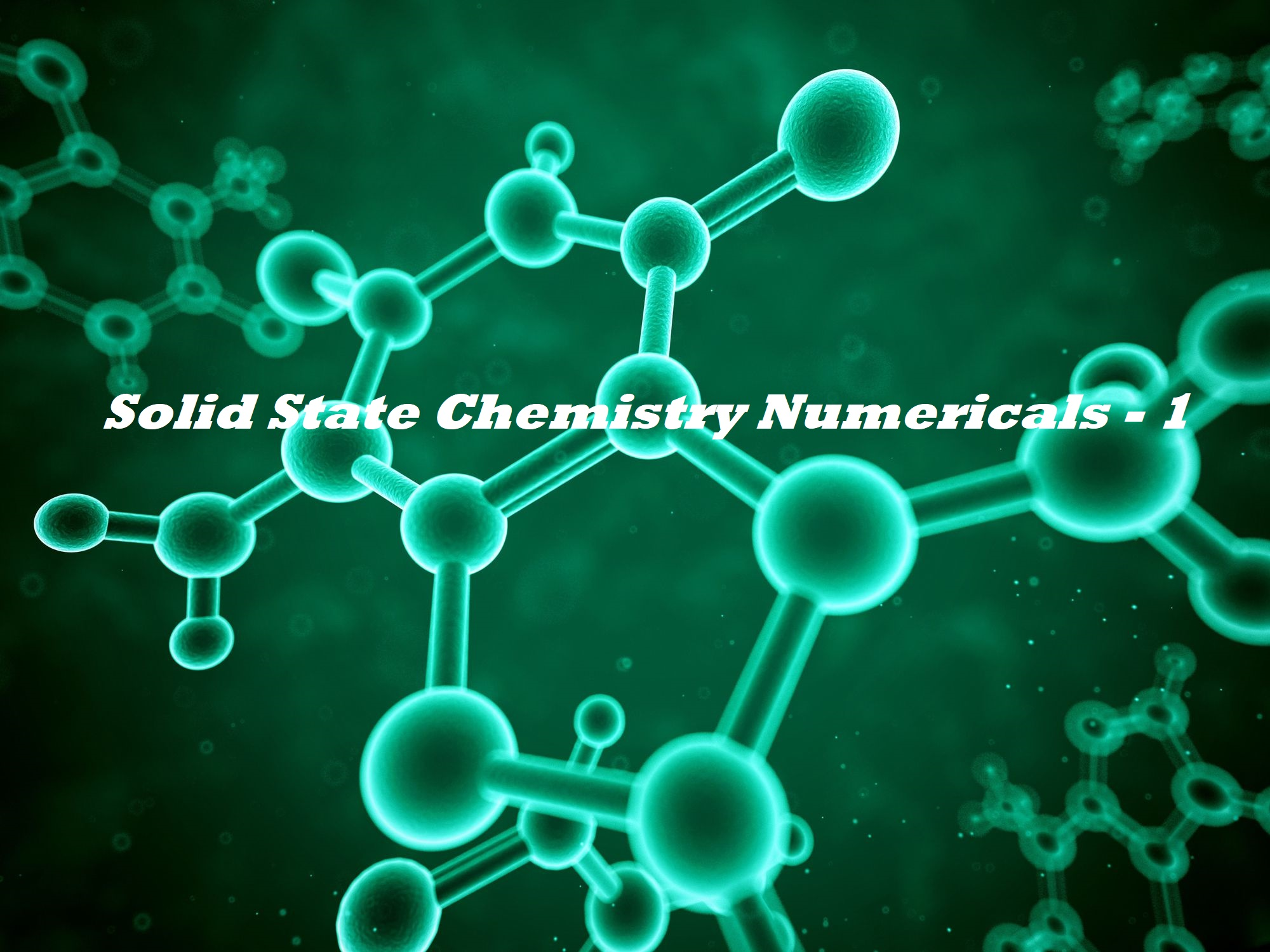Solid State Chemistry Numericals

The post-Solid State Chemistry Numericals contain all important questions related to the topic

Que 1. A solid has a cubic structure in which X atoms are located at the corners of the cube, Y atoms are at the cube centers and O atoms are at the edge centers. What is the formula of the compound?
Ans 1: Atoms of X are present at all the eight corners of the cube. Therefore, each atom of X at the corner makes 1/8 contribution towards the unit cell.
Number of atoms of X per unit cell = 8 × 1/8 = 1
Y atom is present at the body center, thus the contribution of Y towards unit cell = 1 × 1 = 1
O atom is present at each of the edge centers (number of edges of cube = 12) And each O atom present at the edge center will make 1/4 contribution towards the unit cell.
The number of O atoms per unit cell = 12 × 1/4 = 3
The formula of the compound is, therefore XYO3

Que 2. Potassium crystallizes in a body-centered cubic lattice. What is the approximate number of unit cells in 4.0g of potassium? The atomic mass of potassium = 39.
Ans 2. There will be eight atoms at the corners of the cube and one atom at the body center.
\ Number of atoms per unit cell = 8 × 1/8 + 1 × 1 = 2
Number of atoms in 4.0g of potassium = 4/39 × 6.023 × 1023 = 0.618 × 1023
\ Number of unit cells in 4.0g of potassium = 0.618 × 1023/2 = 3.09 ´ 1022

Que 3. Calculate the packing fraction for the Ca unit cell, given that Ca crystallizes in a face-centered cubic unit cell.
Ans 3: One-eight of each corner atom and one-half of each face-centered atom is contained within the unit cell of Ca giving.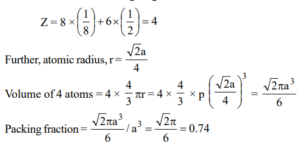Que 4. Gold has a close-packed structure which can be viewed as spheres occupying 0.74 of the total volume. If the density of gold is 19.3 g/cc, calculate the apparent radius of a gold ion in the solid.
Ans 4: Gold has a close-packed structure with a packing fraction value of 0.74. This shows that it has a face-centered cubic cell. The number of ions in a face-centered unit cell is 4.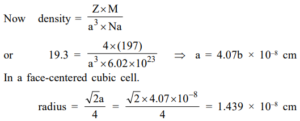Que 5. An element occurs in the bcc structure with a cell edge of 288 pm. The density of metal is 7.2 g cm–3. How many atoms does 208 g of the element contain?
Ans 5: Volume of the unit cell = (288 × 10–10)3 = 23.9 × 10–24 cm3
Volume of 208 g of the element = 208/7.2 = 28.88 cm3
Number of unit cells in 28.88 cm3 = 28.88/23.9 × 10-24 = 12.08 × 1023 unit cells.

Que 6: In a cubic lattice, the closed packed structure of mixed oxides of the lattice is made up of oxide ion one eight of the tetrahedral voids are occupied by divalent ions (A2+) while one-half of the octahedral voids are occupied by trivalent ions (B3+). What is the formula of the oxides?
Ans 6. Let there be n no. of O2– in the crystal.
Octahedra voids = n
Tetrahedral voids = 2n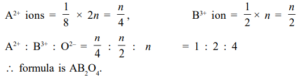Que 7: A compound formed by elements A and B crystallizes in a cubic structure where A atoms are at the corners of a cube and B atoms are at the face center. What is the formula of the compound?
Ans 7: A atom is eight corners of the cube. Hence no. of A atoms = 8 × 1/8  = 1
B atoms are at the face center of six faces.
Hence no. of B atoms 6/2 = 3
The formula is AB3.

Que 8: NH4Cl crystallizes in a body-centered cubic lattice, with a unit cell distance of 387 pm. Calculate:
(a) The distance between the appositively charged ions in the lattice
(b) the radius of the NH4+ ion if the radius of the Cl ion is 181pm.
Ans 8: (a) In a body-centered cubic lattice, oppositely charged ions touch each other along the cross- diagonal of the cube. So, we can write,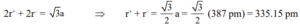(b) Now, since r = 181 pm
We have, r+= (335.15 – 181) pm = 154.15 pm

Que 9: Iron occurs as BCC as well as FCC unit cell. If the effective radius of an atom of iron is 124 pm, compute the density of iron in both these structures.
Ans 9: In a body-centered unit cell, atoms touch each other along the cross diagonal.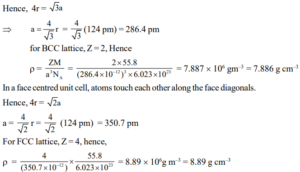Que 10. Silver has a cubic unit cell with a cell edge of 408 pm. Its density is 10.6 g cm–3. How many atoms of silver are there in the unit cell? What is the structure of silver?
Ans 10: Length of the edge of unit cell = 408 pm,
Volume of unit cell = (408 pm)3 = 67.92 × 10–24 cm3
Mass of unit cell = Density × Volume
= 10.6g cm–3 × 67.92 × 10–24 cm3 = 7.20 × 10–24g
Mass of unit cell = No. of atoms in unit cell × Mass of each atom
Now, mass of each atom = Atomic mass/Avogadro number = 108/6.023 × 1023 = 1.79 × 10–22g
Let the unit cell contains ‘n’ atoms, so that Mass of unit cell = n × 1.79 × 10–22 = 7.20 × 10–22
n = 7.20 × 10-22/1.79 1022 = 4.02
\ Number of atoms present in a unit cell = 4 Since the unit cell contains 4 atoms per unit cell, silver has a face-centered cubic lattice.

Que 11. If NaCl is doped with 10–3 mol percent of SrCl2, what is the concentration of cation vacancy?
Ans 11. Concentration of cation vacancies = concentration of Sr2+ ion in the crystal
\ Number of cationic vacancies per mol = 10-3 × 6.022 × 1023 / 100
= 6.023 × 1018 vacancies per mole.

Que 12: A solid A+ B has a NaCl type close-packed structure. If the anion has a radius of 250 pm, what should be the ideal radius of the cation? Can a cation C+ having a radius of 180 pm be slipped into the tetrahedral site of the crystal A+ B? Give the reason for your answer.
Ans 11: As A+ B has a NaCl structure, A+ ions will be present in the octahedral voids. The ideal radius of the cation will be equal to the radius of the octahedral void because in that case it will touch the anions and the arrangement will be closely packed.
Radius of the octahedral void = A r+ = 0.414 × rB = 0.414 × 250 = 103.4 pm
Radius of the tetrahedral site = 0.225 × rB = 0.225 ×250 pm = 56.25 pm
As the radius of the cation C+ (180 pm) is larger than the size of the tetrahedral site, it cannot be slipped into it.